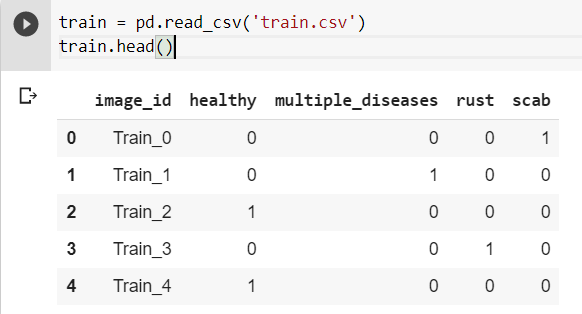# How to use auc roc curve for multi-class model in fast ai

My Dataset Looks Like this a csv file and a bunch of images in a folder :I want calculate `auc_roc_score` for each class as my prediction output.
How to use `auc_roc_score` as metric to get a prediction like thisHere is how to do it:

``````from sklearn.metrics import roc_auc_score
def comp_metric(preds, targs, labels=range(len(LABEL_COLS))):
# One-hot encode targets
targs = np.eye(4)[targs]
return np.mean([roc_auc_score(targs[:,i], preds[:,i]) for i in labels])

def healthy_roc_auc(*args):
return comp_metric(*args, labels=)

def multiple_diseases_roc_auc(*args):
return comp_metric(*args, labels=)

def rust_roc_auc(*args):
return comp_metric(*args, labels=)

def scab_roc_auc(*args):
return comp_metric(*args, labels=)

metrics_=[
AccumMetric(healthy_roc_auc, flatten=False),
AccumMetric(multiple_diseases_roc_auc, flatten=False),
AccumMetric(rust_roc_auc, flatten=False),
AccumMetric(scab_roc_auc, flatten=False),
AccumMetric(comp_metric, flatten=False)
]

learn = cnn_learner(dls, resnet34,metrics=[accuracy,*metrics_])
``````
3 Likes